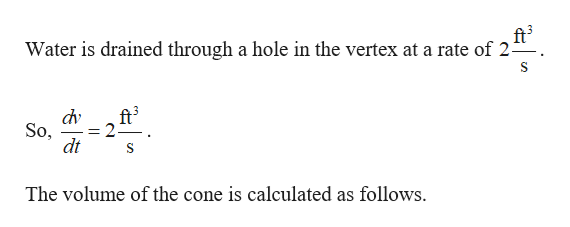# An inverted conical water tank with a height of 12ft and a radius of 6ft ft is drained through a hole in the vertex at a rate of 2ft3/s What is the rate of change of the water depth when the water depth is 3ft​?

Question
314 views

An inverted conical water tank with a height of 12ft and a radius of 6ft ft is drained through a hole in the vertex at a rate of 2ft3/s What is the rate of change of the water depth when the water depth is 3ft​?

check_circle

Step 1
Step 2help_outlineImage Transcriptioncloseft3 Water is drained through a hole in the vertex at a rate of 2 dv ft3 2 So, dt The volume of the cone is calculated as follows fullscreen

### Want to see the full answer?

See Solution

#### Want to see this answer and more?

Solutions are written by subject experts who are available 24/7. Questions are typically answered within 1 hour.*

See Solution
*Response times may vary by subject and question.
Tagged in
MathCalculus

### Other